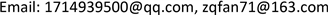﻿ 关于广义Keune符号的若干性质的研究 On Research for Some Properties of General Keune Symbol

Pure Mathematics
Vol.08 No.01(2018), Article ID:23352,7 pages
10.12677/PM.2018.81004

On Research for Some Properties of General Keune Symbol

Lei Wang, Ziqiang Fan

School of Mathematics and Big Data, Anhui University of Science and Technology, Huainan AnhuiReceived: Dec. 18th, 2017; accepted: Jan. 1st, 2018; published: Jan. 8th, 2018ABSTRACT

Keune defined ternary symbol $〈a,b,c〉$ (named by Keune symbol) of commutative ring in 1981, Fan etc generated it to a general ring with identity, and some relations of this symbol are discussed in 2013. In this Paper, we discuss some its properties, these properties are very important to give the presentation of ${K}_{2}$ of stable range one ring.

Keywords:Steinberg Group, K2 Group, Keune Symbol1981年，Keune给出了交换环的三元符号 $〈a,b,c〉$ (我们称之为Keune符号)。2013年，范自强等将Keune符号推广到一般环(含单位元)，并讨论了该符号的一些关系。本文将讨论广义Keune符号的若干性质，这些性质对研究稳定秩1环的 ${K}_{2}$ 群是非常重要的。1. 引言

1) ${x}_{ij}\left(r\right){x}_{ij}\left(s\right)={x}_{ij}\left(r+s\right)$

2) $\left[{x}_{ij}\left(r\right),{x}_{kl}\left(s\right)\right]=\left\{\begin{array}{ll}1,\hfill & i\ne l,j\ne k,\hfill \\ {x}_{ij}\left(rs\right),\hfill & i\ne l,j=k,\hfill \end{array}$

2. 群K(R)的若干关系

${w}_{ij}\left(u\right)={x}_{ij}\left(u\right){x}_{ji}\left(-{u}^{-1}\right){x}_{ij}\left( u \right)$

$a,b,c\in R$$u=a+c-abc\in U\left(R\right),\text{\hspace{0.17em}}v=a+c-cba$ ，令

${W}_{ij}\left(a,b,c\right)={x}_{ij}\left(-a\right){x}_{ji}\left(b\right){x}_{ij}\left(-c\right){x}_{ji}\left({u}^{-1}\left(1-ab\right)\right){x}_{ij}\left(-v\left(1-bc\right)\right)$

${W}_{n}\left(R\right)$ 是由 ${W}_{ij}\left(a,b,c\right)$ 生成的 $S{t}_{n}\left(R\right)$ 的子群，其中 $a,b,c\in R$$a+c-abc\in U\left(R\right)$

$a,b,c\in R$$u=a+c-abc\in U\left(R\right),\text{\hspace{0.17em}}v=a+c-cba$ ，令

${〈a,b,c〉}_{1}={W}_{12}\left(a,b,c\right){w}_{12}\left( v \right)$

${〈a,b,c〉}_{1}$ 为Keune符号。令K(R)是由Keune符号 ${〈a,b,c〉}_{1}$ 生成的 $S{t}_{n}\left(R\right)$ 的子群。在  中给出了如下定理：

1) ${〈a,b,c〉}_{1}={〈c,b,a〉}_{1}^{-1}$

2) ${〈a,b,d〉}_{1}={〈ub,d{u}^{-1},\left(1-ab\right)〉}_{1}$

3) ${〈a,b+c-bac,d〉}_{1}={〈a,b,\left(1-ac\right)d〉}_{1}\cdot {〈a,c,d\left(1-ba\right)〉}_{1}$

4) ${〈a,b,c〉}_{1}={〈a{\gamma }^{-1},\gamma b,c{\gamma }^{-1}〉}_{1}$ ，其中 $\gamma \in U\left(R\right)$

5) $x{〈a,b,c〉}_{1}{x}^{-1}={〈\pi a,b{\pi }^{-1},\pi c〉}_{1}$ ，其中 $x\in K\left(R\right)$$\pi =\phi \left(x\right)$

3. 广义Keune 符号的若干性质

K2) $〈a,b,d〉=〈ub,d{u}^{-1},\left(1-ab\right)〉$

K3) $〈a,b+c-bac,d〉=〈a,b,\left(1-ac\right)d〉\cdot 〈a,c,d\left(1-ba\right)〉$

K4) $〈a,b,c〉=〈a{\gamma }^{-1},\gamma b,c{\gamma }^{-1}〉$ ，其中 $\gamma \in U\left(R\right)$

K5) $〈A,B,C〉〈a,b,c〉{〈A,B,C〉}^{-1}={}^{x}〈a,b,c〉$

$〈a,b〉=〈a-1,-1,b-1〉$$\left\{u,v\right\}=〈u\left(v-1\right),{u}^{-1}〉$ ，我们称 $〈a,b〉$ 为Dennis-Stein符号， $\left\{u,v\right\}$ 为Steinberg符号。

${D}_{R}$ 是由所有 $〈a,b〉$ 生成的 ${K}_{R}$ 的子群， ${S}_{R}$ 是由所有 $\left\{u,v\right\}$ 生成的 ${K}_{R}$ 的子群。若 $u\in U\left(R\right)$ ，记

${}^{u}a=ua{u}^{-1}$${}^{u}\left\{v,w\right\}=\left\{uv{u}^{-1},uw{u}^{-1}\right\}$${}^{u}〈a,b〉=〈ua{u}^{-1},ub{u}^{-1}〉$

D1) ${〈a,b〉}^{-1}=〈b,a〉$

D2) $〈b+c-bac,a〉={}^{1-ba}〈c,a〉\cdot 〈b,a〉$

D3) $〈a,bc〉〈b,ca〉〈c,ab〉=1$

D4) $〈a,b〉〈c,d〉{〈a,b〉}^{-1}={}^{\pi }〈c,d〉$ ，其中 $\pi =\left(1-ab\right){\left(1-ba\right)}^{-1}$

S1) $\left\{u,1-u\right\}=1$

S2) $\left\{u,-u\right\}=1$

S3) $\left\{u,vw\right\}=\left\{u,v\right\}\cdot {}^{v}\left\{u,w\right\}$

S4) $\left\{uv,w\right\}={}^{u}\left\{v,w\right\}\left\{u,w\right\}$

D5) $〈a+c,b〉=〈c{\alpha }^{-1},{}^{\alpha }b〉〈a,b〉$$〈a,b+c〉=〈a,b〉〈{}^{\beta }a,c{\beta }^{-1}〉$ ，其中 $\alpha =1-ab$$\beta =1-ba$

D6) $〈a,0〉=〈0,b〉=1$

S5) $\left\{u,v\right\}\left\{v,u\right\}=1$

S6) $\left\{u,1\right\}=\left\{1,u\right\}=1$

S7) $\left\{u,vw\right\}\left\{v,wu\right\}\left\{w,uv\right\}=1$

S8) $\left\{v,u\right\}={}^{u}\left\{{u}^{-1},v\right\}=\left\{{u}^{-1},{}^{u}v\right\}$

S9) $\left\{u,v\right\}\left\{{u}^{\prime },{v}^{\prime }\right\}{\left\{u,v\right\}}^{-1}={}^{\left[u,v\right]}\left\{{u}^{\prime },{v}^{\prime }\right\}$

1) ${}^{u}\left\{v,v\right\}=\left\{v,v\right\}$ ，其中 $u,v\in U\left(R\right)$

2) $\left\{uv,uv\right\}=\left\{u,u\right\}\left\{v,v\right\}$ ，其中 $u,v\in U\left(R\right)$

3) ${}^{u}\left\{v,w\right\}=\left\{v,w\right\}\left\{{x}^{-1},u\right\}$ ，其中 $x=\left[v,w\right]$$u\in U\left(R\right)$

4) ${}^{u}〈a,b〉=〈a,b〉\left\{{\pi }^{-1},u\right\}$ ，其中 $\pi =\left(1-ab\right){\left(1-ba\right)}^{-1}$$u\in U\left(R\right)$

5) ${}^{u}\rho =\rho \left\{{x}^{-1},u\right\}$ ，其中 $x=\tau \left(\rho \right)$$\rho \in {D}_{R}$$u\in U\left(R\right)$

6) $\left\{\alpha ,\alpha \right\}=\left\{\beta ,\beta \right\}$ ，其中 $a\in R$ 或者 $b\in R$$\alpha =1-ab$$\beta =1-ba$

7) $〈a,b〉=\left\{\alpha ,{\beta }^{-1}\right\}〈-b{\alpha }^{-1},a〉=〈b,-a{\beta }^{-1}〉\left\{{\alpha }^{-1},\beta \right\}$ ，其中 $\alpha =1-ab$$\beta =1-ba$

${}^{u}\left\{v,v\right\}=\left\{uv{u}^{-1},uv{u}^{-1}\right\}=\left\{uv,v{u}^{-1}\right\}\left\{{u}^{-1},uvv\right\}=\left\{uvv,{u}^{-1}\right\}\left\{v,v\right\}\cdot \left\{{u}^{-1},uvv\right\}=\left\{v,v\right\}$

2) 由S3，S4得 $\left\{uv,uv\right\}=\left\{uv,u\right\}\cdot {}^{u}\left\{uv,v\right\}={}^{u}\left\{v,u\right\}\left\{u,u\right\}\left\{v,v\right\}\cdot {}^{u}\left\{u,v\right\}=\left\{u,u\right\}\left\{v,v\right\}$

3) 令 $x=\left[v,w\right]$ ，则 $wv={x}^{-1}vw$$\left\{{u}^{-1},uwv\right\}={}^{{u}^{-1}}\left\{uwv,u\right\}=\left\{wvu,u\right\}$ ，于是

${}^{{x}^{-1}vwu}\left\{u,{u}^{-2}\right\}=\left\{u,{u}^{-2}\right\}=\left\{u,{u}^{-1}\right\}\left\{u,{u}^{-1}\right\}=\left\{u,u\right\}\left\{u,{u}^{-1}\right\}=1$

$\begin{array}{c}{}^{u}\left\{v,w\right\}=\left\{uv{u}^{-1},uw{u}^{-1}\right\}=\left\{uv,w{u}^{-1}\right\}\left\{{u}^{-1},uwv\right\}=\left\{u,vw{u}^{-1}\right\}\left\{v,w\right\}\left\{wvu,u\right\}\\ =\left\{v,w\right\}\cdot {}^{{x}^{-1}}\left\{u,vw{u}^{-1}\right\}\left\{wvu,u\right\}=\left\{v,w\right\}\cdot {}^{{x}^{-1}}\left\{u,vwu{u}^{-2}\right\}\left\{{x}^{-1}vwu,u\right\}\\ =\left\{v,w\right\}\cdot {}^{{x}^{-1}}\left\{u,vwu\right\}\cdot {}^{{x}^{-1}vwu}\left\{u,{u}^{-2}\right\}\cdot {}^{{x}^{-1}}\left\{vwu,u\right\}\left\{{x}^{-1},u\right\}\\ =\left\{v,w\right\}\left\{{x}^{-1},u\right\}\end{array}$

4) 令 $\alpha =1-ab$$\beta =1-ba$ ，则 $\beta ={\pi }^{-1}\alpha$$\left\{{u}^{-1},u\beta {u}^{-1}\right\}={}^{{u}^{-1}}\left\{u\beta {u}^{-1},u\right\}=\left\{\beta ,u\right\}$ ，因此由D3和D4得

$\begin{array}{c}{}^{u}〈a,b〉=〈ua{u}^{-1},ub{u}^{-1}〉=〈ua,b{u}^{-1}〉〈ba{u}^{-1},u〉\\ =〈uab,{u}^{-1}〉〈a,b〉〈{u}^{-1}\left(u\beta {u}^{-1}-1\right),{\left({u}^{-1}\right)}^{-1}〉\\ =〈u\left(\alpha -1\right),{u}^{-1}〉〈a,b〉\left\{{u}^{-1},u\beta {u}^{-1}\right\}\\ =\left\{u,\alpha \right\}〈a,b〉\left\{\beta ,u\right\}=〈a,b〉\cdot {}^{{\pi }^{-1}}\left\{u,\alpha \right\}\left\{{\pi }^{-1}\alpha ,u\right\}\\ =〈a,b〉\cdot {}^{{\pi }^{-1}}\left\{u,\alpha \right\}\cdot {}^{{\pi }^{-1}}\left\{\alpha ,u\right\}\left\{{\pi }^{-1},u\right\}=〈a,b〉\left\{{\pi }^{-1},u\right\}\end{array}$

5) 由S4，S9得 $\left\{w,u\right\}\rho \left\{{x}^{-1},u\right\}=\rho \cdot {}^{{x}^{-1}}\left\{w,u\right\}\left\{{x}^{-1},u\right\}=\rho \left\{{x}^{-1}w,u\right\}$ ，归纳可证得。

6) 因为 $\left\{\beta ,\beta \right\}$ 属于 ${D}_{R}$ 的中心， $\alpha a=a\beta$$b{\alpha }^{-1}={\beta }^{-1}b$ ，由定义3.2和D3得

$\begin{array}{c}\left\{\alpha ,\alpha \right\}=〈\alpha \left(\alpha -1\right),{\alpha }^{-1}〉=〈\alpha ab,{\alpha }^{-1}〉=〈\alpha a,b{\alpha }^{-1}〉〈-b,-a〉\\ =〈a\beta ,{\beta }^{-1}b〉〈-b,-a〉=〈a,b〉〈ba\beta ,{\beta }^{-1}〉〈-b,-a〉\\ =〈a,b〉〈\beta \left(\beta -1\right),{\beta }^{-1}〉〈-b,-a〉\\ =〈a,b〉\left\{\beta ,\beta \right\}〈-b,-a〉=\left\{\beta ,\beta \right\}\end{array}$

7) 在D5中，令 $c=-a$ ，则 $〈0,b〉=〈-a{\alpha }^{-1},\alpha b{\alpha }^{-1}〉〈a,b〉=1$ ，因此由D1有

$\begin{array}{c}〈a,b〉={〈-a{\alpha }^{-1},\alpha b{\alpha }^{-1}〉}^{-1}=〈-\alpha b{\alpha }^{-1},a{\alpha }^{-1}〉=〈-\alpha b{\alpha }^{-1}a,{\alpha }^{-1}〉〈-b{\alpha }^{-1},a〉\\ =〈-\alpha ba{\beta }^{-1},{\alpha }^{-1}〉〈b{\alpha }^{-1},a〉=〈-\alpha \left(\beta -1\right){\beta }^{-1},{\alpha }^{-1}〉〈-b{\alpha }^{-1},a〉\\ =〈\alpha \left({\beta }^{-1}-1\right),{\alpha }^{-1}〉〈-b{\alpha }^{-1},a〉=\left\{\alpha ,{\beta }^{-1}\right\}〈-b{\alpha }^{-1},a〉\end{array}$

$〈a,b〉=〈b,-a{\beta }^{-1}〉\left\{{\alpha }^{-1},\beta \right\}$

K6) 若 $x\in U\left(R\right)$ ，则 $〈xa,b{x}^{-1},xc〉=〈a,b,c〉\left\{v{u}^{-1},x\right\}$

2) $〈a,b,d〉=〈a-p,b{x}^{-1},x\left(d+p-dbp\right)〉\left\{xv,{y}^{-1}{u}^{-1}\right\}\cdot {}^{{y}^{-1}{u}^{-1}}〈p,b〉\left\{{y}^{-1}{u}^{-1},y\right\}\left\{{u}^{-1},v\right\}$ ，其中 $a,b,d,p,{d}^{\prime }\in R$$u=a+d-abd$$v=a+d-dba$$x=1-pb$$y=1-bp$

$p=\left(1-b{d}^{\prime }\right){{u}^{\prime }}^{-1}-\left(1-bd\right){u}^{-1}={{v}^{\prime }}^{-1}\left(\left(1-{d}^{\prime }b\right)u-v\left(1-bd\right)\right){u}^{-1}$ $={{v}^{\prime }}^{-1}\left(d-{d}^{\prime }\right){u}^{-1}={v}^{-1}\left(d-{d}^{\prime }\right){{u}^{\prime }}^{-1}$

$\begin{array}{l}1-p\left(-\left(1-ab\right)v\right)=1+p\left(1-ab\right)v=1+{{v}^{\prime }}^{-1}\left(d-{d}^{\prime }\right){u}^{-1}\left(1-ab\right)v\\ =1+{{v}^{\prime }}^{-1}\left(d-{d}^{\prime }\right)\left(1-ba\right)=1+{{v}^{\prime }}^{-1}\left(\left(v-a\right)-\left({v}^{\prime }-a\right)\right)={{v}^{\prime }}^{-1}v\in U\left(R\right)\end{array}$ ，同理得 $1-\left(-\left(1-ab\right)v\right)p=u{{u}^{\prime }}^{-1}$

$\begin{array}{c}〈p,-\left(1-ab\right)v〉=〈p\left(1-ab\right),-v〉〈-vp,1-ab〉\\ =〈\left(1-{{v}^{\prime }}^{-1}v\right){\left(-v\right)}^{-1},-v〉〈-vp,1-ab〉\\ =\left\{{{v}^{\prime }}^{-1}v,-v\right\}〈-vp,1-ab〉\\ ={}^{{{v}^{\prime }}^{-1}}\left\{v,-v\right\}\left\{{{v}^{\prime }}^{-1},-v\right\}〈-vp,1-ab〉\\ =\left\{{{v}^{\prime }}^{-1},-v\right\}〈-vp,1-ab〉\end{array}$

$\begin{array}{l}-vp-1-ab+\left(vp+1\right)ab\\ =-1-vp\left(1-ab\right)=-vp-1+vpab=-1-vp\left(1-ab\right)\\ =-1-\left(d-{d}^{\prime }\right){{u}^{\prime }}^{-1}\left(1-ab\right)=-\left({v}^{\prime }+\left(d-{d}^{\prime }\right)\left(1-ba\right)\right){{v}^{\prime }}^{-1}=-v{{v}^{\prime }}^{-1}\end{array}$

$〈-vp,1-ab〉=〈-vp-1,-1,-ab〉=〈v{{v}^{\prime }}^{-1},ab{v}^{\prime }{v}^{-1},-vp〉$

$\left\{-v,{{v}^{\prime }}^{-1}\right\}〈a,b,{d}^{\prime }〉\left\{{v}^{\prime },{{u}^{\prime }}^{-1}\right\}=〈v{{v}^{\prime }}^{-1},ab{v}^{\prime }{v}^{-1},-vp〉\cdot {}^{u{{u}^{\prime }}^{-1}}〈a,b,d〉\left\{u{{u}^{\prime }}^{-1}v,{u}^{-1}\right\}$

$\begin{array}{c}{}^{{v}^{\prime }{{u}^{\prime }}^{-1}}\left\{-v,{{v}^{\prime }}^{-1}\right\}\left\{{v}^{\prime },{{u}^{\prime }}^{-1}\right\}={}^{{v}^{\prime }{{u}^{\prime }}^{-1}}\left\{-v,{{v}^{\prime }}^{-1}\right\}\cdot {}^{{v}^{\prime }}\left\{{{u}^{\prime }}^{-1},{{v}^{\prime }}^{-1}\right\}={}^{{v}^{\prime }}\left({}^{{{u}^{\prime }}^{-1}}\left\{-v,{{v}^{\prime }}^{-1}\right\}\left\{{{u}^{\prime }}^{-1},{{v}^{\prime }}^{-1}\right\}\right)\\ ={}^{{v}^{\prime }}\left\{-{{u}^{\prime }}^{-1}v,{{v}^{\prime }}^{-1}\right\}=\left\{{v}^{\prime },-{{u}^{\prime }}^{-1}v\right\}\end{array}$

$〈a,b,{d}^{\prime }〉\left\{{v}^{\prime },-{{u}^{\prime }}^{-1}v\right\}=〈v{{v}^{\prime }}^{-1},ab{v}^{\prime }{v}^{-1},-vp〉\cdot {}^{u{{u}^{\prime }}^{-1}}〈a,b,d〉\left\{u{{u}^{\prime }}^{-1}v,{u}^{-1}\right\}$

$\begin{array}{c}〈v{{v}^{\prime }}^{-1},ab{v}^{\prime }{v}^{-1},-vp〉〈a,b,d〉=〈v{{v}^{\prime }}^{-1}a,b{v}^{\prime }{v}^{-1},-vpu+v{{v}^{\prime }}^{-1}d〉\\ =〈v{{v}^{\prime }}^{-1}a,b{v}^{\prime }{v}^{-1},v{{v}^{\prime }}^{-1}{d}^{\prime }〉={}^{v{{v}^{\prime }}^{-1}}〈a,b,{d}^{\prime }〉\\ =〈a,b,{d}^{\prime }〉\left\{{v}^{\prime }{{u}^{\prime }}^{-1},v{{v}^{\prime }}^{-1}\right\}\end{array}$

$\left\{{v}^{\prime },-{{u}^{\prime }}^{-1}v\right\}=\left\{{v}^{\prime }{{u}^{\prime }}^{-1},v{{v}^{\prime }}^{-1}\right\}\left\{v{u}^{-1},u{{u}^{\prime }}^{-1}\right\}\left\{u{{u}^{\prime }}^{-1}v,{u}^{-1}\right\}=\left\{{v}^{\prime }{{u}^{\prime }}^{-1},v{{v}^{\prime }}^{-1}\right\}\left\{v,{{u}^{\prime }}^{-1}\right\}$

$\begin{array}{c}\left\{{v}^{\prime }{{u}^{\prime }}^{-1},v{{v}^{\prime }}^{-1}\right\}=\left\{{v}^{\prime },{{u}^{\prime }}^{-1}v{{v}^{\prime }}^{-1}\right\}\left\{{{u}^{\prime }}^{-1},v\right\}=\left\{{v}^{\prime },-{{u}^{\prime }}^{-1}v\right\}\cdot {}^{-{{u}^{\prime }}^{-1}v}\left\{{v}^{\prime },-{{v}^{\prime }}^{-1}\right\}\left\{{{u}^{\prime }}^{-1},v\right\}\\ =\left\{{v}^{\prime },-{{u}^{\prime }}^{-1}v\right\}\cdot {}^{-{{u}^{\prime }}^{-1}v}\left({}^{-{{v}^{\prime }}^{-1}}\left\{-{v}^{\prime },{v}^{\prime }\right\}\right)\left\{{{u}^{\prime }}^{-1},v\right\}=\left\{{v}^{\prime },-{{u}^{\prime }}^{-1}v\right\}\left\{{{u}^{\prime }}^{-1},v\right\}\end{array}$

2) 因为 $a-p+x\left(d+p-dbp\right)-\left(a-p\right)b{x}^{-1}x\left(d+p-dbp\right)=uy$

$1-\left(a-p\right)b{x}^{-1}=\left(x-\left(a-p\right)b\right){x}^{-1}=\left(1-ab\right){x}^{-1}$ ，且 $yb=bx$$p{y}^{-1}={x}^{-1}p$

$\left(1-ab\right)\left(1-db\right)=1-\left(a+d-abd\right)b=1-ub$ ，于是有

$\begin{array}{l}〈a-p,b{x}^{-1},x\left(d+p-dbp\right)〉\\ =〈uyb{x}^{-1},x\left(d+p-dbp\right){y}^{-1}{u}^{-1},\left(1-ab\right){x}^{-1}〉\\ =〈uyb,\left(d+p-dbp\right){y}^{-1}{u}^{-1},\left(1-ab\right)〉\\ =〈uyb,d{y}^{-1}{u}^{-1},\left(1-uybp{y}^{-1}{u}^{-1}\right)\left(1-ab\right)〉〈uyb,p{y}^{-1}{u}^{-1},\left(1-ab\right)\left(1-db\right)〉\end{array}$

$\begin{array}{l}={}^{uy{u}^{-1}}〈ub,d{u}^{-1},1-ab〉〈ubx,{x}^{-1}p{u}^{-1},\left(1-ub\right){x}^{-1}x〉\\ ={}^{uy{u}^{-1}}〈a,b,d〉〈ub,p{u}^{-1},\left(1-ub\right){x}^{-1}〉\\ =〈a,b,d〉\left\{v{u}^{-1},uy{u}^{-1}\right\}〈ub,p{u}^{-1}〉\end{array}$

$\begin{array}{l}\left\{xv,{y}^{-1}{u}^{-1}\right\}\cdot {}^{{y}^{-1}{u}^{-1}}〈p,b〉\\ =\left\{xv,{y}^{-1}{u}^{-1}\right\}〈p,b〉\left\{y{x}^{-1},{y}^{-1}{u}^{-1}\right\}\\ =〈p,b〉\cdot {}^{y{x}^{-1}}\left\{xv,{y}^{-1}{u}^{-1}\right\}\left\{y{x}^{-1},{y}^{-1}{u}^{-1}\right\}\\ =〈p,b〉\left\{yv,{y}^{-1}{u}^{-1}\right\}\end{array}$

$〈ub,p{u}^{-1}〉〈p,b〉=〈u,bp{u}^{-1}〉=〈{\left({u}^{-1}\right)}^{-1},{u}^{-1}\left(1-uy{u}^{-1}\right)〉=\left\{uy{u}^{-1},{u}^{-1}\right\}$

$\begin{array}{c}1=\left\{v{u}^{-1},uy{u}^{-1}\right\}\left\{uy{u}^{-1},{u}^{-1}\right\}\left\{yv,{y}^{-1}{u}^{-1}\right\}\left\{{y}^{-1}{u}^{-1},y\right\}\left\{{u}^{-1},v\right\}\\ =\left\{v{u}^{-1},uy{u}^{-1}\right\}\left\{uy{u}^{-1},{u}^{-1}\right\}\cdot {}^{y}\left\{v,{y}^{-1}{u}^{-1}\right\}\left\{y,{y}^{-1}{u}^{-1}\right\}\left\{{y}^{-1}{u}^{-1},y\right\}\left\{{u}^{-1},v\right\}\\ ={}^{u}\left\{{u}^{-1}v,y\right\}\cdot {}^{u}\left\{y,{u}^{-1}\right\}\cdot {}^{y}\left\{v,{y}^{-1}{u}^{-1}\right\}\left\{{u}^{-1},v\right\}\\ ={}^{u}\left\{{u}^{-1}v,y\right\}\cdot {}^{u}\left\{y,{u}^{-1}\right\}\left\{y,v\right\}\left\{v,{u}^{-1}\right\}\left\{{u}^{-1},v\right\}\\ ={}^{u}\left\{{u}^{-1}v,y\right\}\left\{u,y\right\}\left\{y,v\right\}=\left\{v,y\right\}\left\{y,v\right\}\end{array}$

On Research for Some Properties of General Keune Symbol[J]. 理论数学, 2018, 08(01): 22-28. http://dx.doi.org/10.12677/PM.2018.81004

1. 1. Milnor, J. (1971) Introduction to Algebraic K-Theory. Princeton University Press, Princeton.

2. 2. Keune, F. (1972) The K2 of 1-Fold Ring. Springer-Verlag, Berlin, 281-303.

3. 3. Keune, F. (1981) The Another Presentation for the K2 of a Local Domain. Journal of Pure and Applied Algebra, 22, 131-141. https://doi.org/10.1016/0022-4049(81)90055-4

4. 4. Fan, Z.Q., Song, G.T. and Peng, Y.Z. (2013) Relations of Keune Symbols. Comptes Rendus Mathematique, 351, 865- 870. https://doi.org/10.1016/j.crma.2013.11.002

5. 5. Peng, Y.Z., Fan Z.Q. and Song G.T. (2011) On the K2 of a Ring with Stable Range One. Southeast Asian Bulletin of Mathematics, 35, 641-652.